#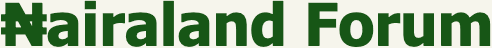Welcome, Guest: Join Nairaland / LOGIN! / Trending / Recent / New
Stats: 2,371,998 members, 5,279,647 topics. Date: Friday, 22 November 2019 at 02:15 PM

## Try Dis If You Are Human - Jokes Etc - Nairaland

 Try Dis If You Are Human by bunmioguns(m): 9:22pm On Apr 23, 2013 6÷2(1+2) = ? Re: Try Dis If You Are Human by Lagusta(m): 9:40pm On Apr 23, 2013 The ans na 1....Oya where my prize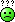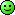Re: Try Dis If You Are Human by outrage: 10:32pm On Apr 23, 2013 9 1 Like Re: Try Dis If You Are Human by bunmioguns(m): 5:04am On Apr 24, 2013 Lagusta: The ans na 1....Oya where my prizeoshi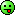Re: Try Dis If You Are Human by bunmioguns(m): 5:06am On Apr 24, 2013 outrage: 9+2000 people including seun, mukina, boko haram like dis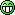Re: Try Dis If You Are Human by Nobody: 2:41am On Jun 01, 2013 Yeah the answer is 9 Re: Try Dis If You Are Human by bunmioguns(m): 7:17am On Jun 01, 2013 Vanneni: Yeah the answer is 9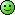Re: Try Dis If You Are Human by Valiantvaliant(m): 10:24am On Jun 03, 2013 the answer is 1 jor Re: Try Dis If You Are Human by oderemo(m): 9:33pm On Jun 03, 2013 what is this rubbish? are we in some jnr class or what.clown shape up, its not by force to post. Re: Try Dis If You Are Human by Nobody: 5:27am On Jun 04, 2013 outrage: 9 Vanneni: Yeah the answer is 9Olodo, u no sabi use BODMAS?2(1+2)=6,6/6=1 Re: Try Dis If You Are Human by Nobody: 8:06am On Jun 04, 2013 ighoosagie: Olodo, u no sabi use BODMAS?2(1+2)=6,6/6=1 What does BODMAS mean? Isn't it bracket first then order, division, multiplication addition and subtraction? That means you treat an equation in that order from left to right? Ok given that we have to deal with what's in the bracket first, We'd have: 1+2 =3 right?----->step one then we treat the division sign next. Meaning we'd have:6/2 which gives 3------>step twoThen we multiply the 3 from step one by the 3 from step two and that gives us 9. Therefore the answer to this equation 6÷2(1+2) = ? is 9.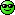Meaning you're a bigger olodo.Re: Try Dis If You Are Human by Nobody: 1:15pm On Jun 04, 2013 Vanneni: What does BODMAS mean? Isn't it division first thenn order, division, multiplication addition and subtraction? That means you treat an equation in that order from left to right? Ok given that we have to deal with what's in the bracket first, We'd have: 1+2 =3 right?----->step one then we treat the division sign next. Meaning we'd have:6/2 which gives 3------>step twoThen we multiply the 3 from step one by the 3 from step two and that gives us 9. Therefore the answer to this equation 6÷2(1+2) = ? is 9.Meaning you're a bigger olodo.BODMAS means B- bracket, O-of(multiplication), D-division, M-multiplicationA-addition, S-subtraction.Re old re u? Dis wud b of great help if u wanna write Ur pry6 common entrance. Re: Try Dis If You Are Human by bunmioguns(m): 1:29pm On Jun 04, 2013 *sits down quietly in a corner and watching vanneni and igboosagie abt exchanging blow* Re: Try Dis If You Are Human by bunmioguns(m): 1:29pm On Jun 04, 2013 Valiantvaliant: the answer is 1 jor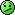Re: Try Dis If You Are Human by bunmioguns(m): 1:30pm On Jun 04, 2013 ode remo: what is this rubbish? are we in some jnr class or what.clown shape up, its not by force to post.Re: Try Dis If You Are Human by bukatyne(f): 4:26pm On Jun 04, 2013 funny, I am getting 76/2(1 + 2)=6 / (2 x 1) + (2 x 2)=6 / 2 + 4using BODMAS,= 6 / 2 = 3 + 4= 76 / 2(1 + 2) is not equal to 6 / (2(1 + 2)) Re: Try Dis If You Are Human by sainty2k3(m): 5:04pm On Jun 04, 2013 Vanneni: What does BODMAS mean? Isn't it division first thenn order, division, multiplication addition and subtraction? That means you treat an equation in that order from left to right? Ok given that we have to deal with what's in the bracket first, We'd have: 1+2 =3 right?----->step one then we treat the division sign next. Meaning we'd have:6/2 which gives 3------>step twoThen we multiply the 3 from step one by the 3 from step two and that gives us 9. Therefore the answer to this equation 6÷2(1+2) = ? is 9.Meaning you're a bigger olodo.You are wrong, if I still remember Bodmas, the first thing is not division , the first thing is the bracket, º°˚˚˚°ºнaĦaнaº°˚˚˚°º‎​ . My ans is 7 jor I agree with bukatyne. Re: Try Dis If You Are Human by Nobody: 5:06pm On Jun 04, 2013 sainty2k3: You are wrong, if I still remember Bodmas, the first thing is not division , the first thing is the bracket, º°˚˚˚°ºнaĦaнaº°˚˚˚°º‎​ . My ans is 7 jor I agree with bukkyanne It was a typo error. I was gon write that the first thing to deal with is the bracket. It means you didn't even read everything I typed coz I started by dealing with the bracket in my main calculation. The correct answer to that equation is 9 joor. Quote me anywhere.Re: Try Dis If You Are Human by Nobody: 5:11pm On Jun 04, 2013 ighoosagie: BODMAS means B- bracket, O-of(multiplication), D-division, A-addition, S-subtraction.Re old re u? Dis wud b of great help if u wanna write Ur pry6 common entrance. It was an error in my typing joor. I was gon type bracket first. You didn't even read my whole write up and you just jumped into conclusion. And where's the M in your bodmasRe: Try Dis If You Are Human by Nobody: 5:15pm On Jun 04, 2013 bunmioguns: *sits down quietly in a corner and watching vanneni and igboosagie abt exchanging blow* I wasn't built to fight those kind of fights. Re: Try Dis If You Are Human by sainty2k3(m): 5:19pm On Jun 04, 2013 Vanneni: It was a typo error. I was gon write that the first thing to deal with is the bracket. It means you didn't even read everything I typed coz I started by dealing with the bracket in my main calculation. The correct answer to that equation is 9 joor. Quote me anywhere.. Ur step 1 is also wrong, u dealt with A addition and not the bracketIn dealing with the bracket the 2(1+2) becomes 2x1 + 2x2 , that means 2+ 4 , so the equation turns 6÷2+4, then u go to division that gives you 3+4 and then 3+4 which is equals to 7 Re: Try Dis If You Are Human by adexsimply(m): 5:44pm On Jun 04, 2013 BODMAS-Bracket Of Division Multiplication Addition Subtraction6÷2(1+2) = dealing with the bracket, 1+2=3,mind you 2(1+2) means 2 of (1+2), which is equivalent to 2 * (3)=6so, we have 6 ÷ 6 =1 Re: Try Dis If You Are Human by adexsimply(m): 5:46pm On Jun 04, 2013 sainty2k3: . Ur step 1 is also wrong, u dealt with A addition and not the bracketIn dealing with the bracket the 2(1+2) becomes 2x1 + 2x2 , that means 2+ 4 , so the equation turns 6÷2+4, then u go to division that gives you 3+4 and then 3+4 which is equals to 7 Nope!! when dealing with the brackets, you have to solve the equation in the bracket first Re: Try Dis If You Are Human by sainty2k3(m): 6:12pm On Jun 04, 2013adexsimply: BODMAS-Bracket Of Division Multiplication Addition Subtraction6÷2(1+2) = dealing with the bracket, 1+2=3,mind you 2(1+2) means 2 of (1+2), which is equivalent to 2 * (3)=6so, we have 6 ÷ 6 =1Ok, I think u are right, but let's look at it this way; when the equation turns 6÷2(3) this actually implies 6÷2x3 which will then be 3x3(division before multiplication) then 3x3=9 . Hmmmm is vanneni right afterall ?adexsimply: BODMAS-Bracket Of Division Multiplication Addition Subtraction6÷2(1+2) = dealing with the bracket, 1+2=3,mind you 2(1+2) means 2 of (1+2), which is equivalent to 2 * (3)=6so, we have 6 ÷ 6 =1Ok, I think u are right, but let's look at it this way; when the equation turns 6÷2(3) this actually implies 6÷2x3 which will then be 3x3(division before multiplication) then 3x3=9 . Hmmmm is vanneni right afterall ? Re: Try Dis If You Are Human by adexsimply(m): 6:19pm On Jun 04, 2013 sainty2k3:Ok, I think u are right, but let's look at it this way; when the equation turns 6÷2(3) this actually implies 6÷2x3 which will then be 3x3(division before multiplication) then 3x3=9 . Hmmmm is vanneni right afterall ?nope..don't ignore the "of" it has precedence over division Re: Try Dis If You Are Human by Nobody: 7:41pm On Jun 04, 2013 adexsimply: nope..don't ignore the "of" it has precedence over division Come and vote for me on the miss NL thread jooor.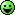Re: Try Dis If You Are Human by Nobody: 7:50pm On Jun 04, 2013 adexsimply: BODMAS-Bracket Of Division Multiplication Addition Subtraction6÷2(1+2) = dealing with the bracket, 1+2=3,mind you 2(1+2) means 2 of (1+2), which is equivalent to 2 * (3)=6so, we have 6 ÷ 6 =1 You're to divide before you multiply says the rule. After dealing with what's in the bracket, you then deal with division and multiplication from the left to the right. Re: Try Dis If You Are Human by albacete(m): 8:17pm On Jun 04, 2013 Vanneni: You're to divide before you multiply says the rule. After dealing with what's in the bracket, you then deal with division and multiplication from the left to the right.Exactly why i voted for u, full of intellect. Say no more guys, Vanneni has said it all. 1 Like Re: Try Dis If You Are Human by Nobody: 8:18pm On Jun 04, 2013 Vanneni: You're to divide before you multiply says the rule. After dealing with what's in the bracket, you then deal with division and multiplication from the left to the right.PEMDASPlease Excuse My Dear Aunt Sally Re: Try Dis If You Are Human by Nobody: 8:19pm On Jun 04, 2013 albacete: Exactly why i voted for u, full of intellect. Say no more guys, Vanneni has said it all.Omo I need more votes o. Lemme go and seek voters on other threads. Re: Try Dis If You Are Human by Nobody: 8:23pm On Jun 04, 2013 Texas.Cowgirl:PEMDASPlease Excuse My Dear Aunt SallyPink Elephants make dandy apple sauce.Re: Try Dis If You Are Human by adexsimply(m): 8:25pm On Jun 04, 2013 Vanneni:Omo I need more votes o. Lemme go and seek voters on other threads.